# Protein Crystallography CourseCourse Homepage   Basic: 1 2 3 4 5 6   Advanced: 7 8 9 10 11 12 13 14 15 16 17 18 19 20 21 22

# Advanced diffraction: waves, interference and complex numbers

This page is a revised version of the first half of basic diffraction theory, aimed at a deeper understanding of the mathematics and physics.

# Waves

Since diffraction arises from the interaction of matter with waves, we have to understand first how to describe waves, both the terminology and the mathematics. Diffraction results from the addition of waves scattered by different objects, so we also have to understand how waves add up and how this reflects the relative positions of the objects scattering them.

We're still treating X-rays as classical waves without worrying about their particle properties. Fortunately, we don't have to bring quantum mechanics into the picture to get a good understanding of diffraction.

## Describing waves

Electromagnetic waves, such as X-rays, vary over time and space. Although there is also a magnetic component that varies perpendicular to the electric field component, it is the variation in the electric field that interests us. That is because, as discussed in the overview, X-rays interact with matter through their interaction with charged particles, particularly electrons.

Whether you look at an electromagnetic wave as a function of position (along the direction of propagation of the wave) at some particular time, or as a function of time, at some particular position, the electric field will have a cosine shape.

Let's consider time first. In crystallographic applications, we can define the phase of the wave to be zero at the origin of time and space, so that it has a peak at the origin at time zero.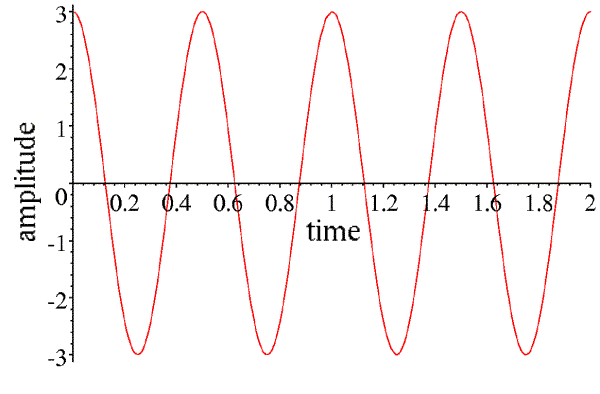This wave can be described by its amplitude (the height of the peaks, in this case 3), and its frequency (how many times it repeats per unit time, in this case 2). Instead of frequency, we can speak of the period of the wave (how long it takes to repeat, in this case half of a time unit), which is the inverse of the frequency. If we call the amplitude A, the time t and the frequency ν, then the equation describing this wave is given by:

A cos(2πνt)

Note that whenever the time is a multiple of the period (or when the product of the time and the frequency is an integer), the argument of the cosine is a multiple of 2π, and the wave repeats.

However, we can also consider a snapshot of this wave at a particular time, as a function of position along its direction of propagation. If we pick time zero, then the phase will be zero and the wave will be at a peak at the origin.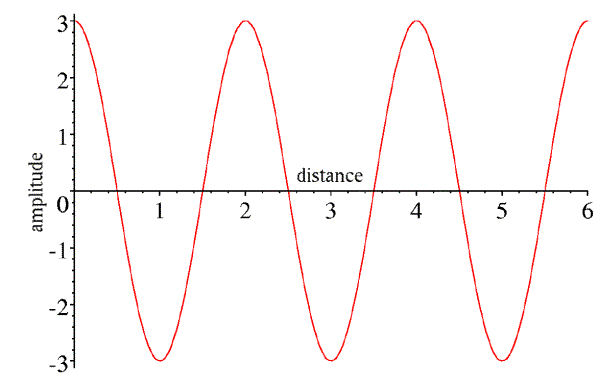Again, this wave has an amplitude of 3. It is also characterised by its wavelength (the distance between peaks, in this case 2). If we call the wavelength λ and the distance along the direction of propagation x, then the expression describing this wave is:

A cos(2πx/λ)

Note that whenever the distance is a multiple of the wavelength, the argument of the cosine is a multiple of 2π, and the wave repeats. Also (and this will become important in just a moment), we could easily replace this expression by A cos(‑2πx/λ), because the cosine is the same for positive and negative arguments.

Now, x was chosen to be along the direction the wave propagates, so if we wait a little while, the wave will move forward. If we wait for a quarter of the period, we will get the following picture: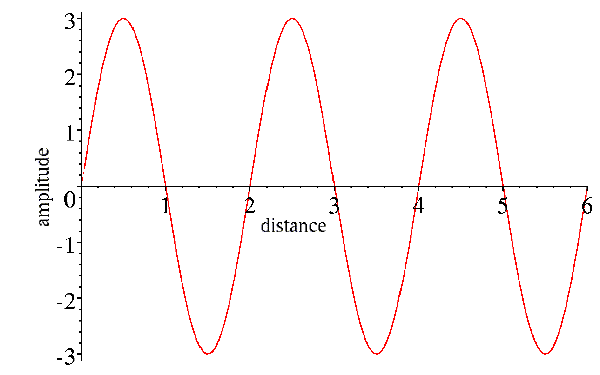Note that the amplitude has dropped off to zero at position zero, as it did after one-quarter of the period in the picture of the wave as a function of time. Now, what we would like to do is to combine the effects of time and position into a single expression for the wave. You can see that we can cancel out the effect of time by moving forward a quarter of a wavelength to get to the peak that started at the origin. So position and time act in opposite senses on the wave, and their effects can be combined by subtracting the effect of position from the effect of time:

A cos[2π(νt-x/λ)]

We can think of the effect of time as having shifted the phase of the wave, so that its peak is no longer at the origin.

It's interesting to think about the size of the units for X-rays. In a typical diffraction experiment, the X-rays have a wavelength of about 1Å, which is one ten-thousand-millionth of a meter. The waves are moving at the speed of light (300 million meters per second), so it takes about 3*10-19 second for the wave to move from peak to peak. Obviously it doesn't make much sense to think about measuring the phase of a photon as it strikes a detector! What matters to us in the diffraction pattern is the relative phase of different diffracted rays. Since all we're interested in is relative phase, then we're free to fix the absolute phase in whatever way we wish. By convention, we fix it so that if a wave is scattered from the origin of the crystal coordinate system, its phase is zero, i.e. it has a peak at the origin. Considered at other points in the crystal, the wave is phase-shifted by the difference in position.

## Waves as vectors

The cosine of an angle is simply the x component of a unit vector after it is rotated by that angle around a unit circle. If the vector is rotated at some constant speed, then its x-value will trace out a cosine wave as a function of time.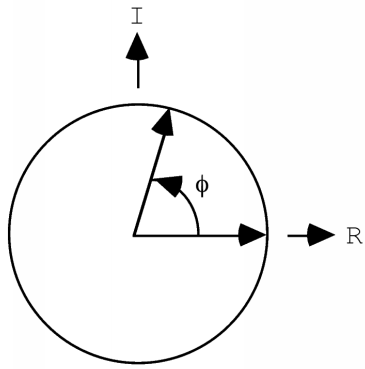If the vector has a length of one, its x-value will trace out a cosine function, but in general it will trace out a wave with an amplitude given by the length of the vector. If we consider a point other than the origin, the wave will start out with a phase other than zero, so it will have a phase shift. If we wanted to worry about waves with different wavelengths or periods, we would have to consider the vectors as rotating at different speeds. But for X-ray crystallography, we're only concerned about the behaviour of a photon, which has a single wavelength. So that allows us to summarise the other properties of the wave as a vector in the plane: the length of the vector represents the amplitude of the wave, and the angle it makes with the horizontal axis represents its phase.

It turns out (as discussed below) that there are great mathematical advantages to considering the vector to be a vector in the complex plane, with real and imaginary components.

If we want to add the expressions for two waves, we can get into some rather nasty trigonometry.

A cos(α+φ1) + B cos(α+φ2)

This is where the vector representation of waves becomes very useful. We can represent the two individual waves as two vectors, one with a length A rotated by an angle φ1 from the horizontal axis, and another with a length B rotated by an angle φ2. We get the wave we want by adding the x component of each of these vectors as they continue to rotate. If we shift the head of the B vector to the tail of the A vector, it doesn't change its x component, even as it rotates, so the x component of the sum of the two vectors defines the sum of the two waves. So we have turned a difficult trigonometric problem into a fairly trivial geometric problem.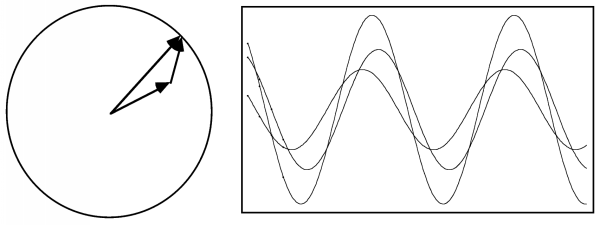The result is a cosine wave with the same wavelength but a different amplitude and phase that are given simply by the sum of two vectors.

## Waves as complex numbers

Complex numbers can be thought of as vectors in the complex plane, with real and imaginary components instead of x and y components as in a real 2D vector. So the addition of waves can be represented as the addition of complex numbers. But complex numbers have additional properties that make them more useful than 2D real vectors for the representation of waves. So wave equations are almost always expressed with complex numbers. Many crystallographers have had little background in complex algebra, so it is probably useful to summarise some concepts here.

### Multiplying complex numbers

One big advantage of complex numbers is that the rotation of a vector can be handled as a multiplication or (equivalently), addition of exponents. We can get a hint of this from the following picture: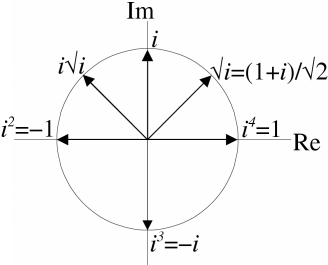We see that the various powers of i are rotated by 90° in the complex plane. Not only that, it turns out that the square root of i is a vector in the complex plane oriented 45° (π/4 radians) from the real axis. And if we multiply i by its square root, it is rotated by 45°. You can verify this easily by doing the math and remembering that i2=-1. We might suspect, then, that multiplication of any two unit vectors in the complex plane will give a new unit vector whose orientation is given by adding the orientation angles of the two vectors being multiplied. Of course, we also need to generalise to consider complex numbers with arbitrary magnitudes.

First we have to consider two ways to represent complex numbers: as the sum of real and imaginary parts, or with polar coordinates:z = a + ib = |z| (cosα + i sinα),
where α is the angle between z and the real axis.

|z| = (a2 + b2)1/2 = [(a + ib)(a - ib)]1/2 = (z z*) 1/2

Some terminology: a = Re(z), or the real part of z, b = Im(z), or the imaginary part of z, i = (-1)1/2, the use of bold font for z indicates that it is a complex number, and z* is called the complex conjugate of z, with the imaginary part negated.

Now we're ready to multiply two complex numbers.

z1 = a1 + ib1 = |z1| (cosα1 + i sinα1)

z2 = a2 + ib2 = |z2| (cosα2 + i sinα2)

z1z2 = |z1||z2| (cosα1 + i sinα1) (cosα2 + i sinα2)

Before going any further, we can see that the product of two complex numbers has a magnitude proportional to (in fact, as we will soon see, equal to) the product of the individual magnitudes, and that the direction is determined by the product of the two corresponding complex numbers of unit length.

(cosα1 + i sinα1) (cosα2 + i sinα2)

= (cosα1 cosα2 + i2 sinα1 sinα2) + i (cosα1 sinα2 + cosα2 sinα1)

= (cosα1 cosα2 - sinα1 sinα2) + i (cosα1 sinα2 + cosα2 sinα1)

= cos(α1 + α2) + i sin(α1 + α2)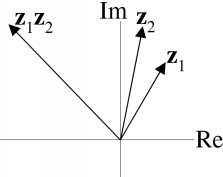To summarise, complex numbers can be represented as a magnitude and phase angle in the complex plane. The product of two complex numbers has a magnitude equal to the product of the magnitudes, and a phase equal to the sum of the phases.

### Euler's equation

As we've seen, the product of the phase components of two complex numbers gives a new phase that is the sum of the two phases. Addition in the context of multiplication might make us think of logarithms and exponentials: to find a product, we can add logarithms. So we might ask what is the logarithm of cosα + i sinα. The (perhaps unintuitive) answer is iα, as seen in a famous equation derived by Euler:

eiα = exp(iα) = cosα + i sinα

If we use Euler's equation to express complex numbers, the addition of the phase angles that takes place in multiplication is obvious.

z1z2 = |z1| exp(iα1) |z2| exp(iα2) = |z1||z2| exp[i(α1 + α2)]

This notation is much tidier than anything we have used so far, which is why it is so useful in dealing with waves.

If you're interested, you can look at two proofs of Euler's equation.

# Diffraction: waves in phase

You can think of X-rays interacting with matter as being scattered (or re-emitted) in all directions from the electrons they encounter. X-rays scattered from different electrons will travel different distances, so they will differ in their relative phases and there will be interference as they add up. They can add up in phase, so that the resulting amplitude is the sum of the individual amplitudes, or out of phase, so that the resulting amplitude is the difference of the individual amplitudes, or anything in between.

## When do waves scatter in phase?

Much of diffraction can be understood qualitatively if we understand when waves scatter in phase. In particular, we can understand why crystals amplify the scattering signal to one we can measure, and why the diffraction pattern is restricted to discrete spots.

### When they have exactly the same pathlength

Diffraction spots are often called reflections, because you can think of the crystal as being composed of thousands of mirrors that reflect the X-rays. These "mirrors" are called Bragg planes.

When light is reflected from a mirror, the angle of incidence (the angle at which it strikes the plane of the mirror) is equal to the angle of reflection. The same is true of Bragg planes, and the reason is that when the angle of incidence is equal to the angle of reflection, light rays hitting the plane (mirror) in phase exit in phase, regardless of where they hit the mirror. The following figure shows why.In this figure, the two incoming light rays are in phase at the line ab. If the lines ad and bc differ in length, they will be out of phase at the line cd. But if those two lines are the same length, they will be in phase. We can see that the lines will be the same length if and only if the angle of incidence is equal to the angle of reflection, by considering two triangles in the figure, abc and cda. The angles abc and cda are both right angles, and the shared side ac must be the same. If one other angle is the same, then the two triangles must be congruent. Now, the angle acb is the angle of incidence, and the angle cad is the angle of reflection. When these angles are the same, then the triangles are indeed congruent and the sides ad and bc must be the same. So the rays reflected off of two points on the plane have identical pathlengths, and remain in phase with one another.

Notice, by the way, that the incoming and outgoing rays differ in direction by a total angle of 2θ. When you are looking at a diffraction pattern, you always have to remember to divide the angle from the direct beam by two to get the angle of incidence, θ!

### When their pathlengths differ by a multiple of the wavelength

If rays reflected from a plane have identical pathlengths, then rays reflected from different planes must have different pathlengths. We can easily work out how far apart the planes must be for the difference in pathlength to be equal to the wavelength of the incoming radiation, so that the scattered rays from the two planes would again be in phase. It turns out that the difference in pathlength depends on the angle of incidence (and reflection). The following figure shows how to work out the relationship, which is called Bragg's law.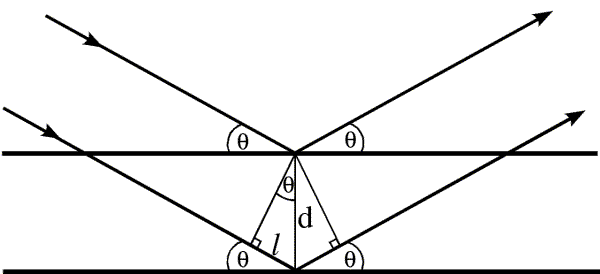The difference in pathlength between the rays reflected from the two planes is twice the distance l. Simple geometry tells us that the upper angle in the little triangle must be θ, because the sum of the angles inside a triangle is 180°, and the other two angles are 90° and 90-θ. Then simple trigonometry tells us that the distance l is equal to d sinθ. For the two rays to be diffracted in phase, twice l must be equal to the wavelength, so we have the relationship:

λ = 2 d sinθ

In fact, the two waves will be in phase if the pathlengths differ by any multiple of the wavelength, so Bragg's law is usually expressed as nλ = 2 d sinθ. However, from the point of view of the information in the diffraction pattern, it makes more sense to choose d so that n is equal to one.

It should also be obvious that, while objects on the planes diffract in phase, objects between the planes will diffract out of phase. The phase shift will be proportional to how far the object is from one plane, as a fraction of the distance to the next plane. So we can see that a single diffraction event tells us about the positions of objects relative to these sets of planes.

In Bragg's law, as the angle increases, d must become smaller for the pathlength to remain equal to one wavelength. We can show this by various common rearrangements of the equation:

sinθ/λ = 1/(2 d)

d = λ/ (2 sinθ)

This is one way of understanding the concept of reciprocal space: the bigger the angle of diffraction, the smaller the spacing to which the diffraction pattern is sensitive.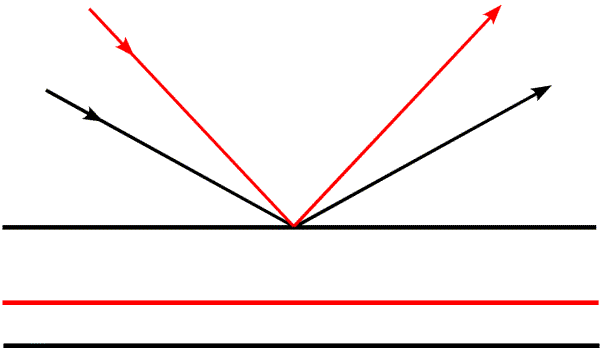In this figure, we see how the spacing from the first to the second plane changes as the angle of incidence changes. The second black plane belongs to the black rays, and the red plane to the red rays. It is useful to think about two limits to the scattering angle: θ=0° and θ=90°. At 0°, the rays are not changed in direction and pathlengths are the same regardless of the positions of objects. The corresponding d-spacing is infinite, which means there is no distance between planes that gives a change in phase, there is no diffraction, and there is no spatial information. When θ is 90°, the waves are reflected straight back at the source. The difference in pathlength is obviously twice the distance between the planes (the waves have to get there and back again). So we are limited to information about spacings equal to half of the wavelength of the radiation we are using. To get higher resolution information, it is necessary to choose a shorter wavelength (which is why we are talking about X-rays instead of visible light, after all).

In the figure above, we have kept the object fixed and changed the angle of the incoming radiation. To observe the same diffraction events, we could also keep the incoming radiation fixed and rotate the object (which is what we do experimentally in an X-ray diffraction experiment.) It is also interesting to consider what happens if you keep both the object and the incoming radiation fixed. Then different diffracted rays correspond to sets of planes that are not parallel to each other, as shown in the following figure: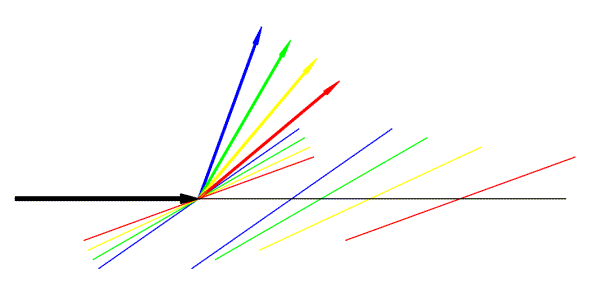In this figure, the large black arrow represents the incoming radiation. Coloured arrows represent different diffracted rays, and a pair of Bragg planes is shown in the same colour.

Let's think about what information we get from a single diffraction event. As mentioned, objects that lie on the Bragg planes will scatter in phase. So if all the objects sat on planes with a particular d-spacing, we would see very strong diffraction for the corresponding direction of scattering. However, if half of the objects sit on the planes, and the other half sit halfway in between, the two sets will scatter out of phase and there will be no diffraction. In general, then, a single point in the diffraction pattern tells us to what extent the objects are concentrated on the corresponding planes. It tells us about the average position of objects in the direction perpendicular to the planes, but nothing about their position in the directions parallel to the planes. This is illustrated in the figure below: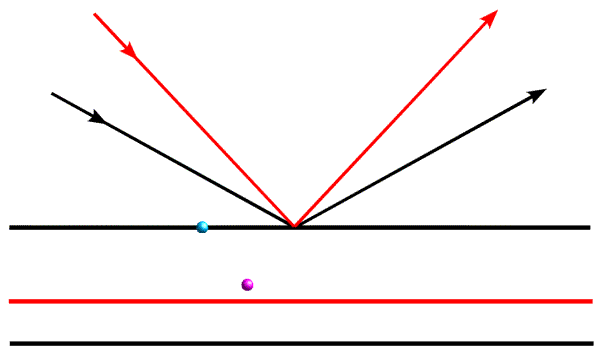When we are considering the diffraction event represented by the black arrows, the blue object and the magenta object will scatter out of phase, so their contributions to the total diffracted wave will cancel out. However, when we look at them with the diffraction event represented by the red arrows, they will add up more nearly in phase.

# Mathematical description of diffraction

We want to describe diffraction mathematically, expressing the amplitude and phase of the diffracted waves in terms of the positions of electrons (atoms) in a crystal, and the directions of the incoming and diffracted X-ray waves.

First we need to describe the incoming X-ray wave. We will assume that the phase of an X-ray diffracted from an electron at the origin of the crystal has a relative phase of zero, so all other phases will be expressed relative to the origin. The direction of the wave can be expressed with a vector in the direction of propagation of the wave. Typically the wave vector is denoted as k. We'll worry in a moment about how to define the magnitude of k.

The incoming X-ray is considered as a plane wave, with peaks in the electric field occurring on planes perpendicular to the wave vector, separated by the wavelength. Interference (as discussed above) is determined by the path length of different X-rays. If we consider diffraction from a particular electron at a position r relative to the origin of the crystal, the following figure shows the pathlength of the X-ray before it reaches that electron, relative to an electron at the origin.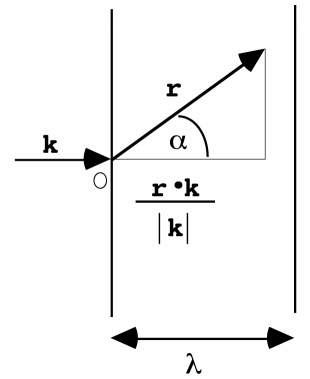What matters is the component of r in the direction of k, which is computed with a dot product between the two vectors. Remember that r·k = |r| |k| cosα; the component of r in the direction of k is given by |r| cosα = r·k / |k|. We divide by λ to find the fraction of a wavelength that is travelled to get to r, giving us r·k / (λ |k|). Now we see that it is most convenient to define the wave vector k to have a magnitude equal to the inverse of the wavelength, so that the denominator disappears. Remembering that position appears with a negative sign in the equation for the phase of a wave, the phase at position r is given by -2πr·k, relative to the origin.

## Phase of diffraction from a single electron

We define the incident beam by the wave vector k0, and the diffracted beam by the wave vector k. As before, both of these have a magnitude equal to the reciprocal of the wavelength. Because the phase of a wave diffracted from the origin is defined to be zero, we can get the phase of a wave diffracted from anywhere else by comparing path lengths. We have to consider both the path to the electron along the incident beam, and the path from the electron along the diffracted beam.

As illustrated in the figure below, the difference in path length (expressed in multiples of the wavelength) is k0·r for the incoming beam, and -k·r for the diffracted beam, so the overall phase of a diffraction event from an electron located at position r is -2π(k0·r-k·r) = 2π(k-k0r (again remembering that minus sign for position).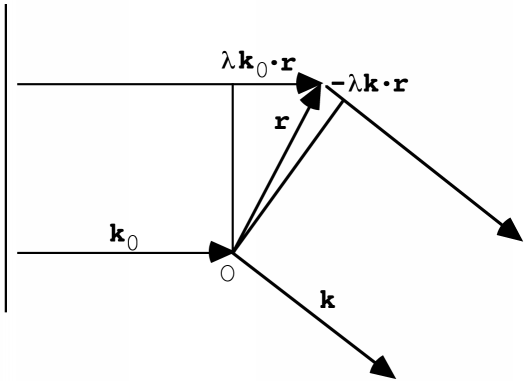Now we define the diffraction vector s = k-k0 and the phase becomes 2πs·r.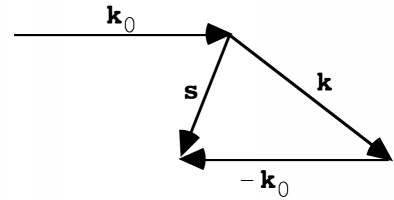So what matters for the phase of diffraction is the component of r in the direction of the diffraction vector s. All points r with the same value of s·r (i.e. satisfying the equation s·r=c) lie on a plane perpendicular to s and diffract with the same phase. The equation for c=0 defines the Bragg plane passing through the origin of the crystal.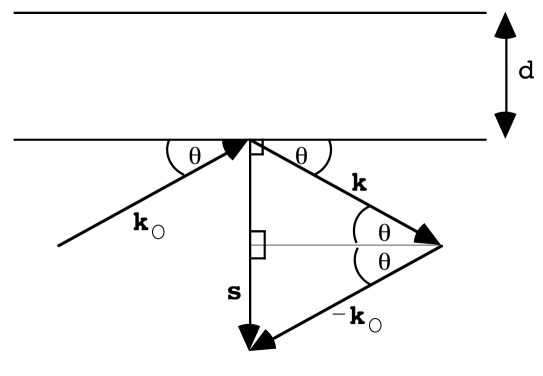We can use this picture to work out Bragg's law again. The phase relative to diffraction from the origin depends on the value of s·r, or the component of r parallel to s. From the figure, we see that

|s| = 2 sinθ |k| = 2 sinθ / λ

When s·r=1, the phase of diffraction is shifted by 2π and the component of r in the direction of s is equal to the d-spacing for this diffraction angle. This means that

|s| d = 1, or |s|=1/d

We can combine the two equations and rearrange to get Bragg's law:

λ = 2 d sinθ

## Physical interpretation of s·r

To get a more intuitive feel for the meaning of the structure factor equation, which will be developed below, it is useful to consider the physical interpretation of s·r. Remember that a dot product can be interpreted as the projection of one vector on the other (the component of one vector that is parallel to the other vector), multiplied by the length of the other vector.

s·r = |s| |r| cosφ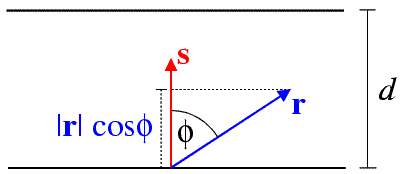In this figure, |r| cosφ is the component of the position vector r in the direction of s, which is perpendicular to the Bragg planes. Since the length of the diffraction vector, |s|, is equal to 1/d, s·r is equal to the fraction of the distance from one Bragg plane to the next that the position vector r has travelled from the origin. (Of course, s·r can be any real number, so it can be greater than one.) We define a wave diffracted from the Bragg plane passing through the origin to have a phase of zero. Waves diffracted from the next Bragg plane have a phase of 2π (which is equivalent to a phase of zero) and, in general, diffraction from any point r will have a phase of 2πs·r.

# Structure factors

## Structure factor from a single electron

The structure factor represents the wave that results from diffraction, which means that it is a complex number with amplitude and phase. To put the structure factor on absolute scale, the diffraction from a single electron at a point is defined as having an amplitude of 1e.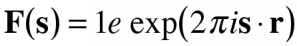The structure factor is often written as a dimensionless number, but you should always remember that it has units of electrons.

If we could measure the phase from a single electron diffraction event, it would only tell us that the electron was located on one of a series of planes separated by the Bragg d-spacing corresponding to the diffraction angle. The higher the angle, the more closely these planes would be spaced.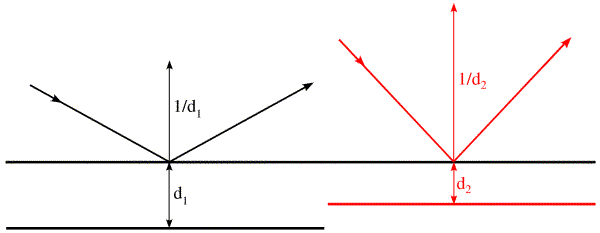## Structure factor from a number of electrons

If diffraction were measured from an object containing several electrons located at different points, the diffraction at each point would be the sum of the waves scattered by each electron. We know now that the sum of waves can be represented most easily by adding complex numbers expressed using Euler's equation, giving us: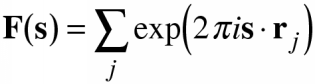The diffraction pattern now gives us information about the relative positions of electrons, because of the interference effects. The bigger the phase differences, the bigger the interference effects, which means that smaller differences in position have measurable effects when the diffraction angle increases. (This is the basis of the concept of resolution.)

## Structure factor from a continuous distribution of electrons

When we go from the discrete to the continuous, we replace a summation by an integral. Instead of an electron at a point, we now have a continuous function, rho(r), the electron density.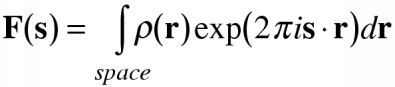This is a very interesting equation. It turns out that the operation that transforms the electron density into a structure factor is something mathematicians know as the Fourier transform. In other words, the diffraction pattern is the Fourier transform of the electron density and we can make use of everything that mathematics has discovered about this function! Most interestingly, the Fourier transform can be reversed.

# Other resources

## Books

T.L. Blundell & L.N. Johnson (1976), "Protein Crystallography", Academic Press: London.

Jan Drenth (1994), "Principles of Protein X-ray Crystallography", Springer-Verlag: New York.

D. Sherwood (1976), "Crystals, X-rays and Proteins", Longman: London.